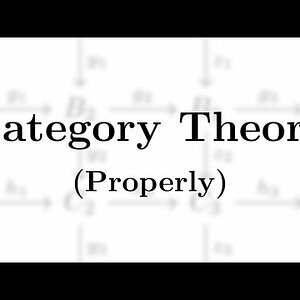# Category theory Definition and 9 Discussions

Category theory formalizes mathematical structure and its concepts in terms of a labeled directed graph called a category, whose nodes are called objects, and whose labelled directed edges are called arrows (or morphisms). A category has two basic properties: the ability to compose the arrows associatively, and the existence of an identity arrow for each object. The language of category theory has been used to formalize concepts of other high-level abstractions such as sets, rings, and groups. Informally, category theory is a general theory of functions.
Several terms used in category theory, including the term "morphism", are used differently from their uses in the rest of mathematics. In category theory, morphisms obey conditions specific to category theory itself.
Samuel Eilenberg and Saunders Mac Lane introduced the concepts of categories, functors, and natural transformations from 1942–45 in their study of algebraic topology, with the goal of understanding the processes that preserve mathematical structure.
Category theory has practical applications in programming language theory, for example the usage of monads in functional programming. It may also be used as an axiomatic foundation for mathematics, as an alternative to set theory and other proposed foundations.

View More On Wikipedia.org
1. ### Towards a new theory of information kinematics

The physical world is highly parallel; many things are happening side by side simultaneously. This is true not only at the macroscopic scales that we can see but as far as we know down to the subatomic scales. This is referred to as the principle of locality , which states that an object is...
2.### A Sensible Introduction to Category Theory

Remember when I used a video with a coconut in the thumbnail to drive a stake through the heart of mathematical structure? Today, in this introduction to the...
3. ### I Functor between the category of Hilbert Space and the category of sets

I have a question that is related to categories and physics. I was reading this paper by John Baez in which he describes a TQFT as a functor from the category nCob (n-dimensional cobordisms) to Vector spaces. https://arxiv.org/pdf/quant-ph/0404040.pdf. At the beginning of the paper @john baez...
4. ### A Structure preserved by strong equivalence of metrics?

Let ##d_1## and ##d_2## be two metrics on the same set ##X##. We say that ##d_1## and ##d_2## are equivalent if the identity map from ##(X,d_1)## to ##(X,d_2)## and its inverse are continuous. We say that they’re uniformly equivalent if the identity map and its inverse are uniformly...
5. ### A Taxonomy of Theories in Theoretical Physics

It goes without saying that theoretical physics has over the years become overrun with countless distinct - yet sometimes curiously very similar - theories, in some cases even dozens of directly competing theories. Within the foundations things can get far worse once we start to run into...
6. ### I System to represent objects in Mathematics

Hi. Usually, Computer Programmers use Flow Charts, Algorithms, or UML diagrams to build a great software or system. In the same manner, in Mathematics, what do Mathematicians use to build a great system that they want to build. Category Theory is at the highest level of abstraction; then...
7. ### Algebra Categories for the Working Mathematician

Hello. I am about to start learning category theory. I keep hearing mixed opinions on the book Categories for the Working Mathematician, by Sanders MacLane (I am aware he is one of the founders of the theory). Some say it's a "must read", and others have called it "outdated." What would seem...
8. ### Understanding the Coproduct in Grp as a Universal Object

Homework Statement Coproducts exist in Grp. This starts on page 71. of his Algebra. Homework Equations [/B] Allow me to present the proof in it's entirety, modified only where it's convenient or necessary for TeXing it. I've underlined areas where I have issues and bold bracketed off my...
9. ### $\hom_A(-,N)$Functor Takes Coproducts to Products

A couple of notes first: 1. \hom_{A}(-,N) is the left-exact functor I'm referring to; Lang gives an exercise in the section preceeding to show this. 2. This might be my own idiosyncrasy but I write TFDC to mean 'The following diagram commutes' 3. Titles are short, so I know that the hom-functor...